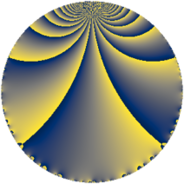# Properties

 Label 50.2.bLevel $50$ Weight $2$ Character orbit 50.b Rep. character $\chi_{50}(49,\cdot)$ Character field $\Q$ Dimension $2$ Newform subspaces $1$ Sturm bound $15$ Trace bound $0$

# Related objects

## Defining parameters

 Level: $$N$$ $$=$$ $$50 = 2 \cdot 5^{2}$$ Weight: $$k$$ $$=$$ $$2$$ Character orbit: $$[\chi]$$ $$=$$ 50.b (of order $$2$$ and degree $$1$$) Character conductor: $$\operatorname{cond}(\chi)$$ $$=$$ $$5$$ Character field: $$\Q$$ Newform subspaces: $$1$$ Sturm bound: $$15$$ Trace bound: $$0$$

## Dimensions

The following table gives the dimensions of various subspaces of $$M_{2}(50, [\chi])$$.

Total New Old
Modular forms 14 2 12
Cusp forms 2 2 0
Eisenstein series 12 0 12

## Trace form

 $$2 q - 2 q^{4} - 2 q^{6} + 4 q^{9} + O(q^{10})$$ $$2 q - 2 q^{4} - 2 q^{6} + 4 q^{9} - 6 q^{11} + 4 q^{14} + 2 q^{16} - 10 q^{19} + 4 q^{21} + 2 q^{24} + 8 q^{26} + 4 q^{31} - 6 q^{34} - 4 q^{36} + 8 q^{39} - 6 q^{41} + 6 q^{44} - 12 q^{46} + 6 q^{49} - 6 q^{51} - 10 q^{54} - 4 q^{56} + 4 q^{61} - 2 q^{64} + 6 q^{66} - 12 q^{69} + 24 q^{71} + 4 q^{74} + 10 q^{76} + 20 q^{79} + 2 q^{81} - 4 q^{84} + 8 q^{86} - 30 q^{89} - 16 q^{91} + 24 q^{94} - 2 q^{96} - 12 q^{99} + O(q^{100})$$

## Decomposition of $$S_{2}^{\mathrm{new}}(50, [\chi])$$ into newform subspaces

Label Dim $A$ Field CM Traces $q$-expansion
$a_{2}$ $a_{3}$ $a_{5}$ $a_{7}$
50.2.b.a $2$ $0.399$ $$\Q(\sqrt{-1})$$ None $$0$$ $$0$$ $$0$$ $$0$$ $$q+iq^{2}+iq^{3}-q^{4}-q^{6}-2iq^{7}+\cdots$$